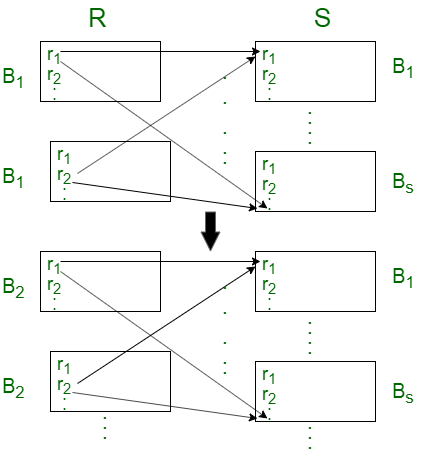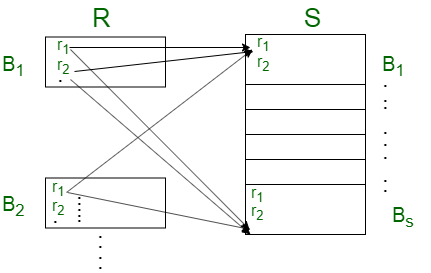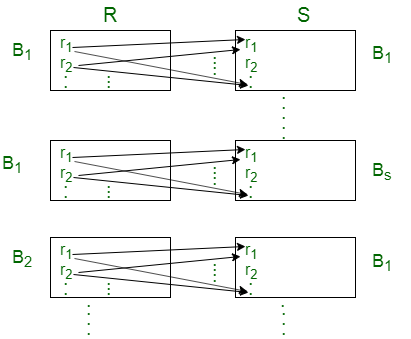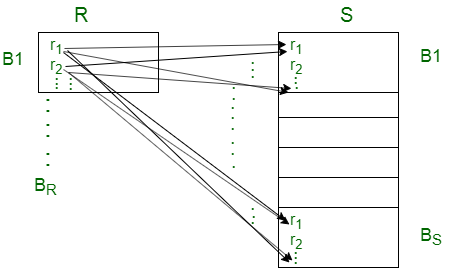# Join algorithms in Database

There are two algorithms to compute natural join and conditional join of 2 relations in database: Nested loop join, and Block nested loop join.

To understand these algorithms we will assume there are two relations, relation R and relation S. Relation R has TR tuples and occupies BR blocks. Relation S has TS tuples and occupies BS blocks. We will also assume relation R is the outer relation and S is the inner relation.

1. Nested Loop Join:
In nested loop join algorithm, for each tuple in outer relation we have to compare it with all the tuples in the inner relation then only the next tuple of outer relation is considered. All pairs of tuples which satisfies the condition are added in the result of the join.

```for each tuple tR in TR do
for each tuple ts in Ts do
compare (tR, ts) if they satisfies the condition
add them in the result of the join
end
end```

This algorithm is called nested join because it consists of nested for loops.

Lets see some cases to understand the performance of this algorithm,

• Case-1: Assume only two blocks of maim memory is available to store blocks from R and S relation.For each tuple in relation R we have to transfer all blocks of relation S and each block of relation R should be transferred only once.
So, the total block transfers needed = TR * BS + BR

• Case-2: Assume one relation fits entirely in the main memory and there is at least space for one extra block.In this case, the blocks of relation S (that is, the inner relation) is only transferred once and kept in the main memory and the blocks of relation R are transferred sequentially. So, all the blocks of both the relation are transferred only once.
So, the total block transfers needed = BR + BS

The relation with lesser number of blocks should be the inner relation to minimize the total number of blocks required in the main memory to complete the join.
That is, min(BR, BS)+1 is the minimum number of blocks in main memory required to join two relations so that no block is transferred more than once.

In nested loop join, more access cost is required to join relations if main memory space allocated for join is very limited.

2. Block Nested Loop Join:
In block nested loop join, for a block of outer relation, all the tuples in that block are compared with all the tuples of the inner relation, then only next block of outer relation is considered. All pairs of tuples which satisfies the condition are added in the result of the join.

```for each block bR in BR do
for each block bs in BS do
for each tuple tR in TR do
for each tuple ts in Ts do
compare (tR, ts) if they satisfies the condition
add them in the result of the join
end
end
end
end ```

Lets look at some similar cases as nested loop join,

• Case-1: Assume only two blocks of maim memory is available to store blocks from R and S relation.For each block of relation R we have to transfer all block of relation S and each blocks of relation R should be transferred only once.
So, the total block transfers needed = BR+ BR * BS

• Case-2: Assume one relation fits entirely in the main memory and there is at least space for one extra block.In this case, total block transfers needed is similar to nested loop join.
Block nested loop join algorithm reduces the access cost compared to nested loop join if main memory space allocated for join is limited.

Related GATE questions:My Personal Notes arrow_drop_upCheck out this Author's contributed articles.

If you like GeeksforGeeks and would like to contribute, you can also write an article using contribute.geeksforgeeks.org or mail your article to contribute@geeksforgeeks.org. See your article appearing on the GeeksforGeeks main page and help other Geeks.

Please Improve this article if you find anything incorrect by clicking on the "Improve Article" button below.

Article Tags :
Practice Tags :

Be the First to upvote.

Please write to us at contribute@geeksforgeeks.org to report any issue with the above content.